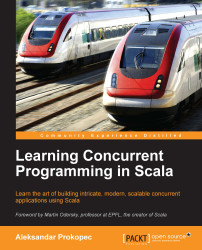•#### Learning Concurrent Programming in Scala

5 (1)5 (1)

#### Overview of this book

Learning Concurrent Programming in ScalaCreditsForewordAcknowledgmentswww.PacktPub.comPrefaceFree Chapter
IntroductionConcurrency on the JVM and the Java Memory ModelAsynchronous Programming with Futures and PromisesData-Parallel CollectionsConcurrent Programming with Reactive ExtensionsSoftware Transactional MemoryActorsConcurrency in PracticeIndex## Exercises

In the following exercises, you will use ScalaSTM to implement various transactional programming abstractions. In most cases, their implementation will closely resemble a sequential implementation, but use transactions. In some cases, you might need to consult external literature or ScalaSTM documentation to correctly solve the exercise.

1. Implement the transactional pair abstraction, represented with the `TPair` class:

```class TPair[P, Q](pinit: P, qinit: Q) {
def first(implicit txn: InTxn): P = ???
def first_=(x: P)(implicit txn: InTxn): P = ???
def second(implicit txn: InTxn): Q = ???
def second_=(x: Q)(implicit txn: InTxn): Q = ???
def swap()(implicit e: P =:= Q, txn: InTxn): Unit = ???
}```

In addition to getters and setters for the two fields, the transactional pair defines the `swap` method that swaps the fields, and can only be called if the types `P` and `Q` are the same.

2. Use ScalaSTM to implement the mutable location abstraction from Haskell, represented with the `MVar` class:

`class...`